# Eleven 11+ Questions: 3. Patterns and SequencesLast Updated: 11 Sep, 2018

In part 3 of our 11+ Questions series we are going to look into patterns and sequences - a very typical exam question - using an example question taken from a sample paper sent out by one of the most top schools in London.

11+ examiners love patterns and sequences. If there is one question that is almost guaranteed to turn up in the exam, it’s the sequences one. Sometimes these will be pretty simple, such as asking for the next step in a pattern that goes 4, 8, 12, … At other times, though, the patterns can be fiendishly difficult, and we’re looking at one such example today.

Today’s question comes from recent sample paper from one of London’s top day schools, and it’s designed to push the best candidates to the limit. In answering it I’m also going to show you some tricks with these sequences to help you get the answer as quickly as possible.

So let’s have a look at question number 3!

## Question 3: A pattern is made using black and white squares. The first four patterns are shown below: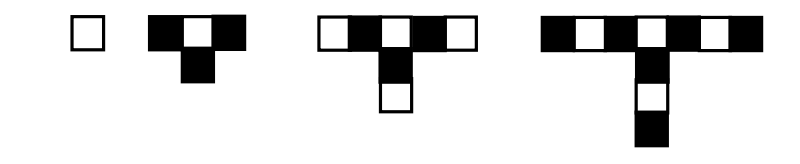a) Complete the table below: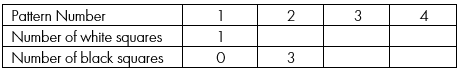Ok, so we have a sequence here that is gradually expanding, and adding on three squares each time.

It’s fairly straightforward to answer this first question. We know how many white squares there are in pattern number 2 because we can count them – there is 1. Likewise there are 4 white squares in pattern number 3. In pattern number 4 the number of white squares will stay the same, so 4 would be the correct answer. Likewise, in pattern number 3 there are the same number of black squares as in pattern number 2 (we can count them), and there are going to be the same number in pattern number 4. So our finished table will look like this: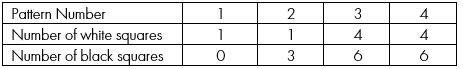That was easy! Now let’s move on to the next question:

b) How many black squares will there be in the 8th pattern?

Right, so now things are going to get slightly more complicated. Now, there are different ways we can approach this question. The easiest approach though is simply to visualise the next couple of patterns.

We can see that with every two patterns we get either 3 more black squares or 3 more white squares:We can then count on and see that in the eighth pattern there will be 12 black squares, because there were 6 in the Pattern 4 and 9 in Pattern 6.

Good! Again, not too difficult. Let’s now look at questions ‘c’, ‘d’ and ‘e’.

c) How many squares will there be in total in the 19th pattern?

d) Which pattern number has 31 white squares and 33 black squares?

e) Sam draws a pattern like the ones above and says, «there are 96 black squares and 95 white squares in my pattern.» How can you tell that he must have
miscounted?

In order to answer these three questions we need to start being a little more  sophisticated in how we approach the problem. We need a method that allows us to calculate how many white squares or black squares there are going to be at a given point in the pattern.

We can approach this either by using algebra (for more advanced candidates) or by simply visualising ahead in the pattern. I’m going to demonstrate both approaches.

## Approach 1 – counting ahead

Let’s begin with question ‘c’ – ‘How many squares in total will there be in the 19th pattern?’

Let’s calculate how many white squares there are in pattern 19 and then how many black squares. We’ll add our answers together to get the correct answer. The first way to do this is to see that the white squares increase by 3 with every odd pattern number. So for PN 1 there is 1 white square, for PN 3 there are 4, PN 5 there are 7 white squares etc. Since 19 is an odd number we can simply visualise forward, adding as we go: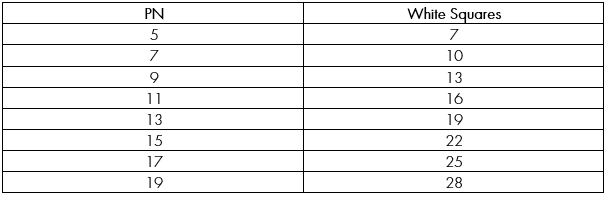So we know that there are going to be 28 white squares in the 19th pattern. But how many black squares will there be?

Let’s look at the relationship between the Pattern Number and the black squares. We can see that the black squares increase by three with every odd number, thus: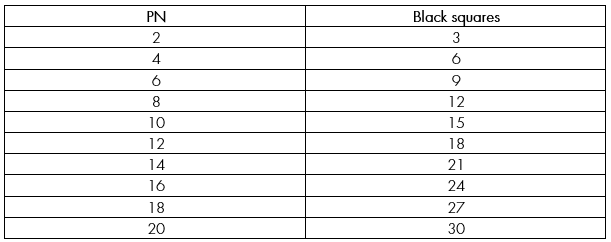Ok, so now we have a problem. Does the 19th Pattern Number have 27 or 30 black squares? The original table will tell us the answer: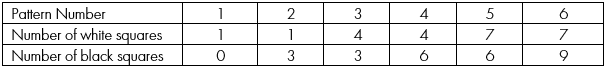We can see that on any particular even number PN (such as 4) the black squares are the same on both the even number and the following odd number. So to take 4 as an example, on PN 4 there are 6 black squares, and on PN 5 there are also 6.

This means that we can actually make a table like this: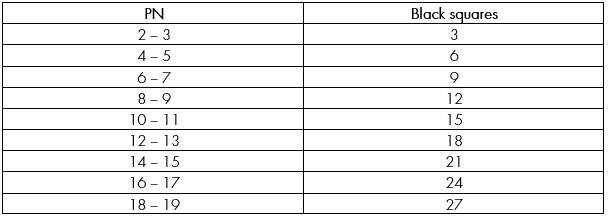There are therefore 27 black squares and 28 whites squares in the 19th pattern, giving us a total of 55 squares overall.

Now let’s look at question ‘d’.

d) Which pattern number has 31 white squares and 33 black squares?

To get the answer to this we can keep counting ahead in the same way. By using the black squares table above we can see that the PN with 33 black squares will be either 22 or 23. The question then is which PN has 31 white squares? Let’s look at our white squares table again, but this time add on one row: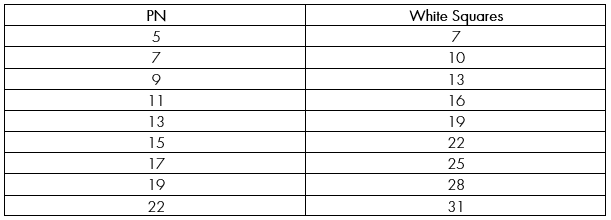And there’s our answer! The pattern number with 31 white squares and 33 black squares is PN 22.

Finally, question ‘e’:

e) ‘Sam draws a pattern like the ones above and says, «there are 96 black squares and 95 white squares in my pattern.» How can you tell that he must have
miscounted?’

To answer this final question we need to use algebra, and so before we attempt it I’m going to show you an algebraic method of doing questions ‘c’ and ‘d’.

## Approach 2 – using an equation to solve a sequence

Let’s begin this section with a look at a very basic pattern:If we want to find the result for any pattern number, we need to find the equation that governs the sequence. We do this by following a few basic steps.

• Call the pattern number, the bit that goes 1,2,3,4 etc. ‘n’.
• Call the result ‘x’
• Begin by writing ‘x =’

The point is that something happens to n, the pattern number, to turn it into x.

So what is happening to n? Well, here we can see that with each step in the sequence x goes up by 3, and if there is a regular difference in steps we know that the three times table is at play. So we can get on the way to writing our equation by putting down:

x = 3n

Now, if this were our equation then the pattern would look like this: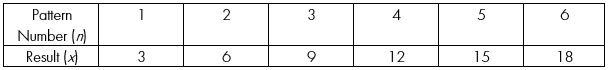It doesn’t, because the result (or x) is always one more than we expect it to be (I.e. instead of 3 we get 4, instead of 12 we get 13 etc.). So we can finish our equation by writing:

x = 3n + 1

Here we have an equation that explains how we get the result from the pattern number. What we have to do now is find such an equation for both our black squares and our white squares.

Let’s go back to our original sequence: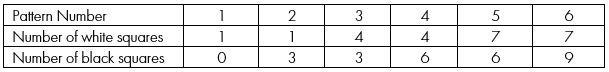There are actually two sequences here. In one sequence something happens to the pattern number that changes the number of white squares. In the other sequence something happens to the pattern number that changes the number of black squares. So let’s try and find  each individually.

• Finding the equation for the white squares

Let’s look at just the white squares.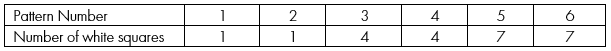We can see that the number of white squares goes up by three with each odd number. So instead of thinking in regular integers, 1,2,3,4 etc., we actually need to think in terms of odd numbers:But hang on, how do we know what number is the ‘6th odd number’ for example? Well, we can work it out by looking at a sequence of odd numbers and working out how to get the sequence.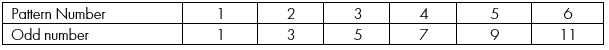We know that the 2 times table is involved because the numbers go up by 2 each time. So we can write:

x = 2n

But if that were true by itself our sequence would look like this: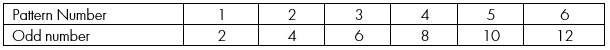So instead we need to subtract by one each time: x = 2n – 1.

So, for example, let’s ask what is the 13th odd number? We use the equation, replacing ‘n’ with 13. The result is this:

x = 2 x 13 – 1. So the 13th odd number is 25.

So now let’s look at this table again…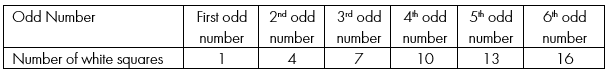…but change it to look like this: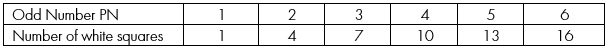Can we find the equation? We certainly can. The 3 times table is involved because there’s a difference of 3 each time, so our equation is this:

x = 3n – 2

So how do we use all of this information to find the number of white squares?

This is what we do:

• Identify what odd number we are on.

Let’s say we’re solving question ‘c’ and we want to know how many white squares there are in sequence 19. Firstly we need to know what odd number 19 is. We already have a sequence for working out odd numbers, so let’s plug it into the sequence.

19  = 2n – 1.

We solve the equation, and see that n = 10. Therefore 19 is the 10th odd number.

• So we now take 10 (for tenth) and plug it into our second equation, x = 3n – 2 .

x = 3 (10) – 2. Therefore x is 28 and there are 28 white squares in the 19th pattern number.

We also need to understand how many white squares there are on the even numbers, but if we look back at our original white squares pattern…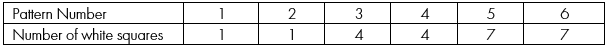…we can see that there are the same number of white squares on the even number following the odd number. That means that there will be the same number of white squares on PN 2 as there are on 1, or the same number on PN 98 as there are on 97 etc.

So we have a method for finding the number of white squares. Great! Now let’s look at the black squares.In this pattern, we can see that the number changes with every even number, and that the number remains the same on the following odd number.

So on pattern number 2 we have 3 black squares, and we also have 3 on pattern number 3.

We use a similar method as before to calculate the number of black squares.

• Work out which even number we are on.

Let’s think of question ‘c’ again and work out how many black squares there are in pattern number 19. We know that there will be the same number of black squares in pattern number 18 as 19, so let’s use 18.

Firstly, what even number is 18? We can calculate even numbers with this equation:

x = 2n.

So, for example, the first even number is x = 2 (1) which is 2. The fifth even number is x = 2 (5) which is 10.

Let’s put 18 into this sequence.

18 = 2n. Therefore 18 is the 9th even number.

• Now have a look at these two tables: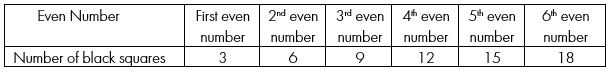…but change it to look like this: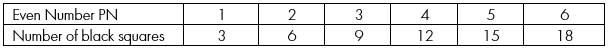So now we need to find an equation for this sequence. We can see that the number of black squares goes up by 3 with each step, so we can write the equation like this:

x = 3n.

We know that the 18th step in the original sequence is the 9th even number. So if we put 9 into this sequence…

x = 3 (9)

We see that there are 27 black squares in the 18th pattern number, and therefore in the 19th pattern number too.

Now let’s look at question ‘d’.

1. Which pattern number has 31 white squares and 33 black squares?

Let’s begin with the white squares.

Let’s put 31 into our white squares equation:

x = 3n – 2  becomes

31 = 3n – 2

Therefore the pattern number with 31 white squares is the eleventh odd number. Now let’s put 11 into our odd number equation:

x = 2n – 1 becomes

x = 2 (11) – 1

Therefore the 11th odd number is 21.

So we know that PN 21 has 31 white squares, but so does PN 22. So our answer could be 21 or 22.

Let’s look at the black squares.

Firstly let’s put 33 into our black squares equation:

x = 3n becomes

33 = 3n. Therefore the 11th even number has 33 black squares.

To find the 11th even number we simply multiply 11 by 2, which is 22. Therefore the answer to question ‘d’ is 22.

Finally, question ‘e’.

‘Sam draws a pattern like the ones above and says, «there are 96 black squares
and 95 white squares in my pattern.» How can you tell that he must have
miscounted?’

So, all we need to do is put the numbers into our equations and prove that it doesn’t work. Let’s begin this time with the black squares.

Let’s put 96 into our even number equation:

96 = 3n, therefore our pattern number is even number 32.

Multiply that by 2 and we get 64 as the pattern number for Sam’s question.

PN 64 will have the same number of white squares as PN 63, so let’s put PN 63 into our white squares equations and see if we get 95 white squares.

Firstly we need to work out what odd number 63 is, so we put it into the equation:

63 = 2n – 1. This results in the odd number 32. We now put 32 into our white squares equation:

x = 3n – 2

Therefore x = 3 (32) – 2. The answer to this is 94, not 95, and therefore we know that Sam has miscounted!

A very, very difficult question, but not impossible. Keep an eye out on the UKSC blog for another post specifically about sequences and how to make equations for them. We’ll see you next time for our next 11+ question!

Educational Consultancy and School Placement Success stories Private tuition